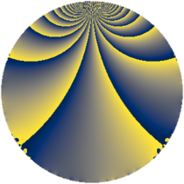# Properties

 Label 3969.1.qLevel $3969$ Weight $1$ Character orbit 3969.q Rep. character $\chi_{3969}(2186,\cdot)$ Character field $\Q(\zeta_{6})$ Dimension $8$ Newform subspaces $1$ Sturm bound $504$ Trace bound $0$

# Related objects

## Defining parameters

 Level: $$N$$ $$=$$ $$3969 = 3^{4} \cdot 7^{2}$$ Weight: $$k$$ $$=$$ $$1$$ Character orbit: $$[\chi]$$ $$=$$ 3969.q (of order $$6$$ and degree $$2$$) Character conductor: $$\operatorname{cond}(\chi)$$ $$=$$ $$21$$ Character field: $$\Q(\zeta_{6})$$ Newform subspaces: $$1$$ Sturm bound: $$504$$ Trace bound: $$0$$

## Dimensions

The following table gives the dimensions of various subspaces of $$M_{1}(3969, [\chi])$$.

Total New Old
Modular forms 128 24 104
Cusp forms 32 8 24
Eisenstein series 96 16 80

The following table gives the dimensions of subspaces with specified projective image type.

$$D_n$$ $$A_4$$ $$S_4$$ $$A_5$$
Dimension 8 0 0 0

## Trace form

 $$8 q + 4 q^{4} + O(q^{10})$$ $$8 q + 4 q^{4} - 8 q^{16} + 8 q^{22} - 4 q^{25} + 4 q^{46} + 4 q^{58} - 16 q^{64} - 4 q^{67} + 4 q^{79} + O(q^{100})$$

## Decomposition of $$S_{1}^{\mathrm{new}}(3969, [\chi])$$ into newform subspaces

Label Dim $A$ Field Image CM RM Traces $q$-expansion
$a_{2}$ $a_{3}$ $a_{5}$ $a_{7}$
3969.1.q.a $8$ $1.981$ $$\Q(\zeta_{24})$$ $D_{12}$ $$\Q(\sqrt{-7})$$ None $$0$$ $$0$$ $$0$$ $$0$$ $$q+(\zeta_{24}^{7}-\zeta_{24}^{9})q^{2}+(-\zeta_{24}^{2}+\zeta_{24}^{4}+\cdots)q^{4}+\cdots$$

## Decomposition of $$S_{1}^{\mathrm{old}}(3969, [\chi])$$ into lower level spaces

$$S_{1}^{\mathrm{old}}(3969, [\chi]) \cong$$ $$S_{1}^{\mathrm{new}}(189, [\chi])$$$$^{\oplus 4}$$$$\oplus$$$$S_{1}^{\mathrm{new}}(441, [\chi])$$$$^{\oplus 3}$$$$\oplus$$$$S_{1}^{\mathrm{new}}(1323, [\chi])$$$$^{\oplus 2}$$